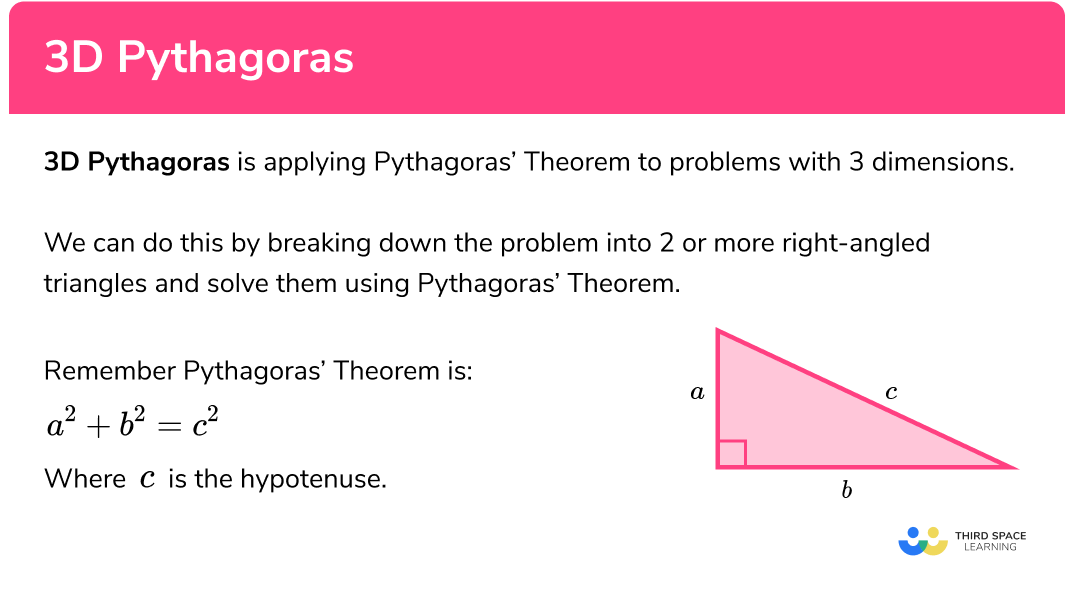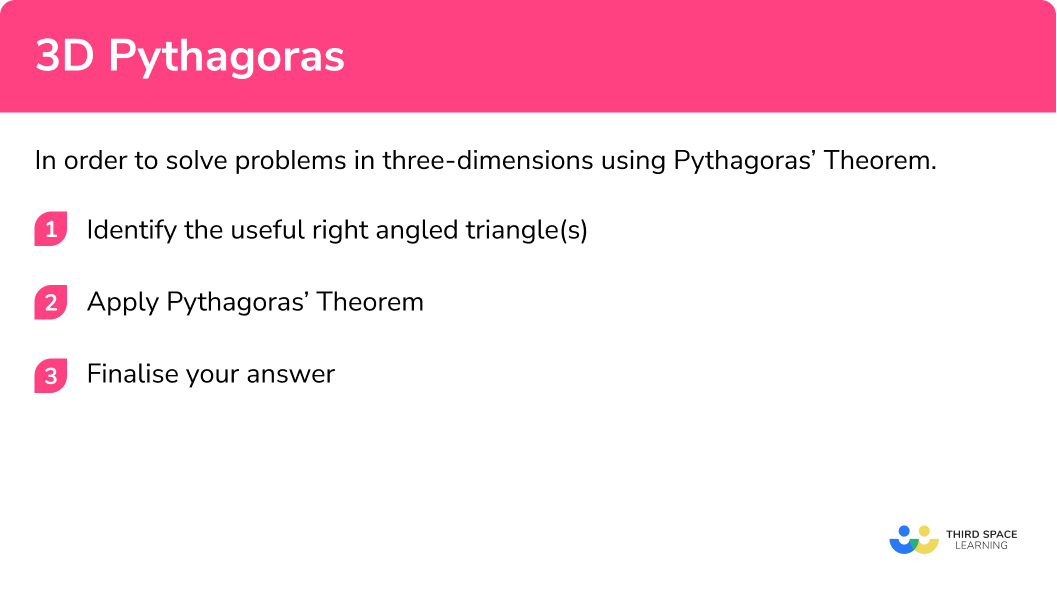# 3D Pythagoras

Here we will learn about 3D Pythagoras including how to apply Pythagoras’ theorem in three dimensional situations.

There are also 3D Pythagoras worksheets based on Edexcel, AQA and OCR exam questions, along with further guidance on where to go next if you’re still stuck.

## What is 3D Pythagoras?

3D Pythagoras is applying Pythagoras’ theorem to problems with 3 dimensions.

We can do this by breaking down the problem into 2 or more right-angled triangles and solve them using Pythagoras’ theorem.

Remember Pythagoras’ theorem is:

$a^2+b^2=c^2$

Where c is the hypotenuse.

### What is 3D Pythagoras?### 3D Pythagoras worked example

Let’s take a look at an example:

We are going to find the length AG in the cuboid ABCDEFGH.

We can see that we can make a right angled triangle ACG which we can use to work out AG.

In order to use Pythagoras’ theorem we need to know two sides of the triangle. So in order to work out the longest side AG we first need to work out one of the shorter sides AC.

Let’s call this side x and redraw this triangle.

We can see that the side we have labeled x forms the diagonal line of the base of the cuboid.

We can see that the triangle ABC is a right angled triangle so we can use Pythagoras’ theorem to calculate x.

$AC=\sqrt{10^2 + 4^2} = 2\sqrt{29} = 10.7703…$

So,

$AG=\sqrt{10.7703…^2 + 6^2} = \sqrt{152}=2\sqrt{38} = 12.328…$

So the required length is 12.3 cm (correct to 1 decimal place).

### 3D Pythagoras for cuboids

We can also use a special 3D Pythagoras’ Theorem for cuboids where x, y, z are the sides of a cuboid and s is the longest diagonal in the cuboid.

$x^2+y^2+z^2=s^2$

E.g

\begin{aligned} &s^{2}=10^{2}+4^{2}+6^{2} \\ &s=\sqrt{10^{2}+4^{2}+6^{2}} \\ &s=12.3 \mathrm{~cm} \quad(1 . \mathrm{d} \cdot \mathrm{p}) \end{aligned}

If angles and lengths are involved in the problem, we may also need to use trigonometry.

## How to solve problems using 3D Pythagoras

In order to solve problems in three-dimensions using Pythagoras’ theorem:

1. Identify the useful right angled triangle(s).
2. Apply Pythagoras’ Theorem.

### How to solve problems using 3D Pythagoras## 3D Pythagoras examples

### Example 1: cones

Find the slant length of the cone with height 10 cm and radius 8 cm. Give your answer correct to 1 decimal place.

1. Identify the useful right angled triangle(s).

We can draw a diagram of the cone and look for the right-angled triangle. The slant length, l, is the hypotenuse of the right-angled triangle with the radius and the height being the adjacent sides to the right-angle.

2Apply Pythagoras’ Theorem.

\begin{align*} a^2 + b^2 &= c^2 \\ 8^2 + 10^2 &= l^2\\ l^2 &= 8^2+10^2\\ l^2 &= 64 + 100\\ l^2 &=164\\ l &= \sqrt{164} \\ \end{align*}

$l = \sqrt{164}=12.8062…$

The slant length of the cone is 12.8 cm (to 1 decimal place).

This is useful as we need the slant length in order to find the curved surface area of a cone.

### Example 2: cones

Find the height of the cone with radius 12 cm and slant length 17 cm. Give your answer correct to 1 decimal place.

Identify the useful right angled triangle(s).

Apply Pythagoras’ Theorem.

### Example 3: cuboids

Here is a cuboid ABCDEFGH. Find the length AG. Give your answer correct to 1 decimal place.

Identify the useful right angled triangle(s).

Apply Pythagoras’ Theorem.

### Example 4: cuboids

Here is a cuboid ABCDEFGH. Find the length BH. Give your answer correct to 1 decimal place.

Identify the useful right angled triangle(s).

Apply Pythagoras’ Theorem.

### Example 5: pyramids

The diagram shows a square-based pyramid ABCDX.

$AB=12 cm$
$AX=BX=CX=DX=17 cm$

The point M is the midpoint of AB.

Calculate the length MX.

Identify the useful right angled triangle(s).

Apply Pythagoras’ Theorem.

### Example 6: pyramids

The diagram shows a square-based pyramid ABCDX.

$AB=20 cm$
$AX=BX=CX=DX=30 cm$

M is the centre of the square base ABCD.

Calculate the length MX.

Identify the useful right angled triangle(s).

Apply Pythagoras’ Theorem.

### Common misconceptions

• Three dimensions can be difficult to visualise

It can be tricky to identify where right angles are on a 2D diagram of a 3D shape. You may find it useful to use a box to help you to visualise it, such as an old tissue box or a shoe box.

• Use all of the digits on the calculator screen

It is very easy to make a mistake at the end with rounding.  Make sure you use all of the digits on the calculator screen and only round your final answer at the end of the question. Rounding too soon will lose accuracy.

• Lengths of sides do not have to be whole numbers

Lengths can be decimals, fractions or even irrational numbers such as surds
E.g.
\sqrt{2} .

### Practice 3D Pythagoras questions

1. Find the slant length of the cone with height 15 cm and radius 10 cm . Give your answer correct to 1 decimal place.

18.0 cm18.1 cm11.2 cm11.1 cml=\sqrt{15^2 + 10^2}=18.02775…

2. Find the radius of the cone with height 19 cm and slant length 23 cm . Give your answer correct to 1 decimal place.

12.9 cm13.0 cm29.8 cm29.9 cml=\sqrt{23^2 – 19^2}=12.96148…

3. Here is a cuboid ABCDEFGH. Find the length AG. Give your answer correct to 1 decimal place.13.1 cm12.4 cm12.5 cm13.2 cmAG=\sqrt{10^2 + 3^2 + 8^2}=\sqrt{173}=13.1529…

4. Here is a cuboid ABCDEFGH. Find the length BH. Give your answer correct to 1 decimal place.20.2 cm20.3 cm12.4 cm12.5 cmBH=\sqrt{7^2 + 6^2 + 18^2}=\sqrt{409}=20.22374…

5. The diagram shows a square-based pyramid ABCDX.
AB=10 cm
AX=BX=CX=DX=18 cm
The point M is the midpoint of AB.
Calculate the length MX.17.2 cm14.9 cm17.3 cm15.0 cmMX=\sqrt{18^2 – 5^2}=\sqrt{299}=17.29161…

6. The diagram shows a square-based pyramid ABCDX.
AB=40 cm
AX=BX=CX=DX=50cm
M is the centre of the square base ABCD.
Calculate the length MX.41.3 cm26.5 cm26.4 cm41.2 cmAC =\sqrt{40^2 + 40^2} = \sqrt{3200}= 40\sqrt{2} = 56.5685…

So,
AM=\frac{1}{2}AC=20\sqrt{2}=28.28427…\\ MX=\sqrt{50^2 – 28.28427…^2} = 10\sqrt{17} = 41.2310…

### 3D Pythagoras GCSE questions

1. Rectangle ABCD is the horizontal base of a triangular prism ABCDEF.
AE = BE\\ AB = 10 cm\\ AE = 17 cm\\ BC = 25 cmWork out the volume of the prism. Give your answer correct to 3 significant figures.

(5 marks)

h^2=17^2 – 5^2
for starting to use Pythagoras to find the height

(1)

h=\sqrt{17^2 – 5^2} = 2\sqrt{66} = 16.24807…
for finding the height of the triangle

(1)

Area = \frac{1}{2}\times 10 \times 2\sqrt{66} = 10\sqrt{66}=81.24038…
for finding the area of the triangle

(1)

Volume = 10\sqrt{66} \times 25 = 2031.0096…
for finding the volume of the triangular prism

(1)

Volume = 2030 cm^3
for the final volume

(1)

2. The diagram shows a box in the shape of a cuboid ABCDEFGH.AB = 12.5 cm\\ AE = 6.7 cm\\ BC = 3.8 cm

A string runs diagonally across the box from D to F.

Calculate the length of the string DF.

(3 marks)

DB=\sqrt{12.5^2 + 3.8^2} = 13.06483…
for using Pythagoras to find DB

(1)

DF=\sqrt{13.06483…^2 + 6.7^2} = 14.68264…
for using Pythagoras to find DF

(1)

DF = 14.7 cm
for the final length

(1)

3. A cone has a radius of 10 cm .
The curved surface area of the cone is 800 cm^2 .
Find the volume of the cone.(5 marks)

\pi \times 10 \times l=800
for setting up the equation to find the slant length

(1)

l=\frac{800}{10\pi}=\frac{80}{\pi}
for finding the slant length

(1)

h=\sqrt{(\frac{80}{\pi})^2-10^2}=23.419128…
for finding the height

(1)

V=\frac{1}{3}\times \pi \times 10^2 \times 23.419128… = 2452.445…
for finding the volume

(1)

V=2452.445… = 2450 cm^3

(1)

## Learning checklist

You have now learned how to:

• Use and apply Pythagoras’ theorem to solve problems in three dimensions
• Solve multi-step problems

## Still stuck?

Prepare your KS4 students for maths GCSEs success with Third Space Learning. Weekly online one to one GCSE maths revision lessons delivered by expert maths tutors.

Find out more about our GCSE maths tuition programme.